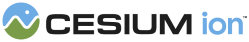#Plane

#### new Cesium.Plane(normal, distance) engine/Source/Core/Plane.js 34

A plane in Hessian Normal Form defined by
```ax + by + cz + d = 0
```
where (a, b, c) is the plane's `normal`, d is the signed `distance` to the plane, and (x, y, z) is any point on the plane.
Name Type Description
`normal` Cartesian3 The plane's normal (normalized).
`distance` number The shortest distance from the origin to the plane. The sign of `distance` determines which side of the plane the origin is on. If `distance` is positive, the origin is in the half-space in the direction of the normal; if negative, the origin is in the half-space opposite to the normal; if zero, the plane passes through the origin.
##### Example:
``````// The plane x=0
const plane = new Cesium.Plane(Cesium.Cartesian3.UNIT_X, 0.0);``````

### Members

#### staticconstant Cesium.Plane.ORIGIN_XY_PLANE : Plane engine/Source/Core/Plane.js 295

A constant initialized to the XY plane passing through the origin, with normal in positive Z.

#### staticconstant Cesium.Plane.ORIGIN_YZ_PLANE : Plane engine/Source/Core/Plane.js 303

A constant initialized to the YZ plane passing through the origin, with normal in positive X.

#### staticconstant Cesium.Plane.ORIGIN_ZX_PLANE : Plane engine/Source/Core/Plane.js 311

A constant initialized to the ZX plane passing through the origin, with normal in positive Y.

#### distance : number engine/Source/Core/Plane.js 65

The shortest distance from the origin to the plane. The sign of `distance` determines which side of the plane the origin is on. If `distance` is positive, the origin is in the half-space in the direction of the normal; if negative, the origin is in the half-space opposite to the normal; if zero, the plane passes through the origin.

#### normal : Cartesian3 engine/Source/Core/Plane.js 54

The plane's normal.

### Methods

#### static Cesium.Plane.clone(plane, result) → Plane engine/Source/Core/Plane.js 254

Duplicates a Plane instance.
Name Type Description
`plane` Plane The plane to duplicate.
`result` Plane optional The object onto which to store the result.
##### Returns:
The modified result parameter or a new Plane instance if one was not provided.

#### static Cesium.Plane.equals(left, right) → boolean engine/Source/Core/Plane.js 277

Compares the provided Planes by normal and distance and returns `true` if they are equal, `false` otherwise.
Name Type Description
`left` Plane The first plane.
`right` Plane The second plane.
##### Returns:
`true` if left and right are equal, `false` otherwise.

#### static Cesium.Plane.fromCartesian4(coefficients, result) → Plane engine/Source/Core/Plane.js 119

Creates a plane from the general equation
Name Type Description
`coefficients` Cartesian4 The plane's normal (normalized).
`result` Plane optional The object onto which to store the result.
##### Returns:
A new plane instance or the modified result parameter.

#### static Cesium.Plane.fromPointNormal(point, normal, result) → Plane engine/Source/Core/Plane.js 83

Creates a plane from a normal and a point on the plane.
Name Type Description
`point` Cartesian3 The point on the plane.
`normal` Cartesian3 The plane's normal (normalized).
`result` Plane optional The object onto which to store the result.
##### Returns:
A new plane instance or the modified result parameter.
##### Example:
``````const point = Cesium.Cartesian3.fromDegrees(-72.0, 40.0);
const normal = ellipsoid.geodeticSurfaceNormal(point);
const tangentPlane = Cesium.Plane.fromPointNormal(point, normal);``````

#### static Cesium.Plane.getPointDistance(plane, point) → number engine/Source/Core/Plane.js 158

Computes the signed shortest distance of a point to a plane. The sign of the distance determines which side of the plane the point is on. If the distance is positive, the point is in the half-space in the direction of the normal; if negative, the point is in the half-space opposite to the normal; if zero, the plane passes through the point.
Name Type Description
`plane` Plane The plane.
`point` Cartesian3 The point.
##### Returns:
The signed shortest distance of the point to the plane.

#### static Cesium.Plane.projectPointOntoPlane(plane, point, result) → Cartesian3 engine/Source/Core/Plane.js 175

Projects a point onto the plane.
Name Type Description
`plane` Plane The plane to project the point onto
`point` Cartesian3 The point to project onto the plane
`result` Cartesian3 optional The result point. If undefined, a new Cartesian3 will be created.
##### Returns:
The modified result parameter or a new Cartesian3 instance if one was not provided.

#### static Cesium.Plane.transform(plane, transform, result) → Plane engine/Source/Core/Plane.js 207

Transforms the plane by the given transformation matrix.
Name Type Description
`plane` Plane The plane.
`transform` Matrix4 The transformation matrix.
`result` Plane optional The object into which to store the result.
##### Returns:
The plane transformed by the given transformation matrix.
Need help? The fastest way to get answers is from the community and team on the Cesium Forum.